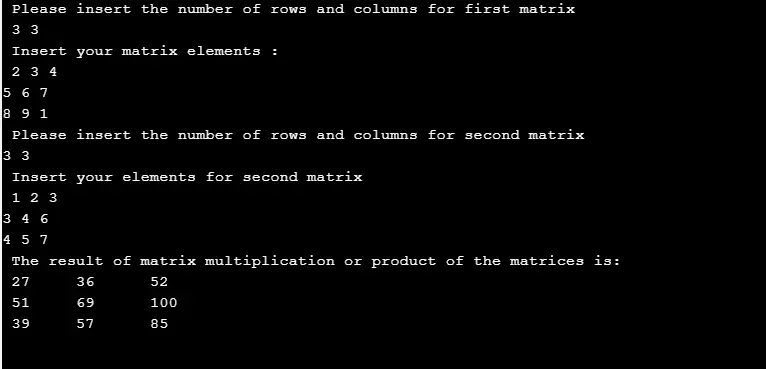# Matrix Multiplication in C

Matrix multiplication is another important program that makes use of the two-dimensional arrays to multiply the cluster of values in the form of matrices and with the rules of matrices of mathematics. In this C program, the user will insert the order for a matrix followed by that specific number of elements. This same thing will be repeated for the second matrix.

In case, the orders of the matrices are such that they cannot get multiplied by each other, then this program will generate an error message.

## Matrix Multiplication Program in C

Example Program:
``````#include<stdio.h>

int main(void)
{
int c, d, p, q, m, n, k, tot = 0;
int fst, sec, mul;

printf(" Please insert the number of rows and columns for first matrix \n ");
scanf("%d%d", &m, &n);

printf(" Insert your matrix elements : \n ");
for (c = 0; c < m; c++)
for (d = 0; d < n; d++)
scanf("%d", &fst[c][d]);

printf(" Please insert the number of rows and columns for second matrix\n");
scanf(" %d %d", &p, &q);

if (n != p)
printf(" Your given matrices cannot be multiplied with each other. \n ");
else
{
printf(" Insert your elements for second matrix \n ");

for (c = 0; c < p; c++)
for (d = 0; d < q; d++)
scanf("%d", &sec[c][d] );

for (c = 0; c < m; c++) {
for (d = 0; d < q; d++) {
for (k = 0; k < p; k++) {
tot = tot + fst[c][k] * sec[k][d];
}
mul[c][d] = tot;
tot = 0;
}
}

printf(" The result of matrix multiplication or product of the matrices is: \n ");
for (c = 0; c < m; c++) {
for (d = 0; d < q; d++)
printf("%d \t", mul[c][d] );
printf(" \n ");
}
}
return 0;
}``````
Program Output:### Explanation

First of all, you must include the standard input-output header file, which is the basis for C programming. Then you have to declare and define the main() function. Within the scope of main() function, you have to first declare some integer variables - c, d, p, q, m, n, k, tot = 0 followed by some 2D integer arrays (which will act like matrices in your program) - fst, sec, mul. Then use a print statement to instruct your user for providing or giving input for a number of rows and columns of the first matrix.

Inserting all the elements one by one in your array needs for loop, followed by a scanf().

``````for (c = 0; c < m; c++)
for (d = 0; d < n; d++)
scanf("%d", &fst[c][d] );
``````

This is a nested for loop which will take values for "m" number of rows and "n" number of columns, i.e., it will iterate "m" and "n" number of times to feed values in the fst[][] array. Again, use another print statement to instruct your user for providing or giving input for a number of rows and columns of the second matrix.

``````for (c = 0; c < p; c++)
for (d = 0; d < q; d++)
scanf("%d", &sec[c][d] );
``````

Same nested loops are used again to feed values to your second array sec[][]. Now the condition is checked whether the number of columns in the first matrix is equal to the number of rows in the second matrix. If this condition satisfies, you have to write the logic for multiplication. This will need nested of nested for loop.

``````for (c = 0; c < m; c++) {
for (d = 0; d < q; d++) {
for (k = 0; k < p; k++) {
tot = tot + fst[c][k] * sec[k][d];
}

mul[c][d] = tot;
tot = 0;
}
}``````

Here the three loops have been used which stores the multiplicative value of fst[][] and sec[][] in the variable tot and this adding of multiplicative values will continue till it traverses all the values of the array. At the same time store every calculated value of tot in the array mul[][] which will store the resultant multiplication. Now you have to print the resultant 2D array using nested for loop.

`````` printf(" The result of matrix multiplication or product of the matrices is: \n ");
for (c = 0; c < g; c++) {
for (d = 0; d < bb; d++)
printf( " %d \t", mul[c][d]);``````